In the verge of coronavirus pandemic, we are providing FREE access to our entire Online Curriculum to ensure Learning Doesn't STOP!

# Ex.6.4 Q1 Squares and Square Roots - NCERT Maths Class 8

Go back to  'Ex.6.4'

## Question

Find the square root of each of the following numbers by division method.

(i) $$2304$$

(ii) $$4489$$

(iii) $$3481$$

(iv) $$529$$

(v) $$3249$$

(vi) $$1369$$

(vii) $$5776$$

(viii) $$7921$$

(ix) $$576$$

(x) $$1024$$

(xi) $$900$$

(xii) $$900$$

Video Solution
Squares And Square Roots
Ex 6.4 | Question 1

## Text Solution

What is Known?

Perfect squares

What is unknown?

Square root by using division method.

Reasoning:

When a number is large, even the method of trading the square root by prime factorization becomes lengthy and difficult, so the division method is used.

Steps

(i)

The square root of $$2304$$ is calculated as follows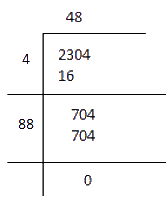Since remainder is zero and number of digits and left in the given number. Therefore,

$$\sqrt {2304}= 48$$

(ii)

The square root of $$4489$$ is calculated as follows.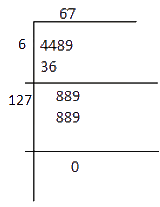\begin{align}\sqrt {4489} = 67\end{align}

(iii)

The square root of $$3481$$ is calculated as follows.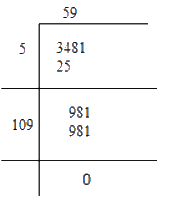$$\sqrt {3841}= 59$$

(iv)

The square root of $$529$$ is calculated as follows.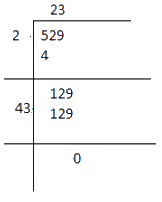$\sqrt {529}= 23$

(v)

The square root of $$3249$$ is calculated as follows.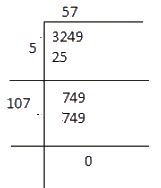$\sqrt {3249}= 57$

(vi)

The square root of $$1369$$ is calculated as follows.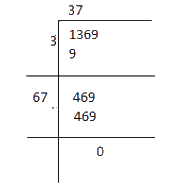$\sqrt {1369}= 37$

(vii)

The square root of $$5776$$ is calculated as follows.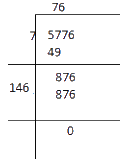$\sqrt {5776}= 146$

(viii)

The square root of $$7921$$ is calculated as follows.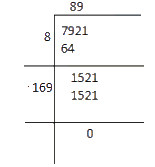$\sqrt {7921}= 89$

(ix)

The square root of $$576$$ is calculated as follows.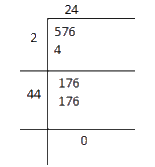$\sqrt {576}= 24$

(x)

The square root of $$1024$$ is calculated as follows.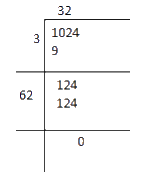$\sqrt {1024}= 32$

(xi)

The square root of $$3136$$ is calculated as follows.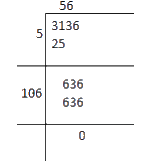$\sqrt {3136}= 56$

(xii)

The square root of $$900$$ is calculated as follows.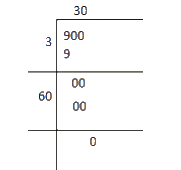$\sqrt {900}= 30$

Learn from the best math teachers and top your exams

• Live one on one classroom and doubt clearing
• Practice worksheets in and after class for conceptual clarity
• Personalized curriculum to keep up with school